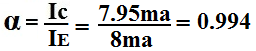﻿ How to Calculate α (alpha) of a transistor# How to Calculate α (alpha) of a Transistor

α (Alpha), the factor by which emitter current is multiplied to yield collector current, can be calculated in two ways.

1st Way to Calculate Alpha α
If the β of the transistor is known, α can be calculated very easily by the formula:Example
If β of a transistor is 100, calculate the α of the transistor:2nd Way to Calculate Alpha α
If the collector current, Ic, and the emitter current, Ie, are known, then α can be calculated by the following formula:Example
If the collector current Ic= 7.95ma and the emitter current Ie=8ma, then alpha calculates out to be:Related Resources